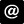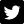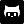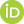### Update!

A new version of permute has been released and some of the functionality described in this post is out of date.

In a previous post I introduced the permute package and the function shuffle(). In that post I got as far as replicating R’s base function sample(). Here I’ll briefly outline how shuffle() can be used to generate restricted permutations.

shuffle() has two arguments: i) n, the number of observations in the data set to be permuted, and ii) control, a list that defines the permutation design describing how the samples are permuted.

control is a list, and for complex permutation designs. As a result, several convenience functions are provided that make it easier to specify the design you want. The main convenience function is permControl() which if passed no arguments populates an appropriate control object with defaults that result in free permutation of observations.

Several types of permutation can be produced by functions in permute:

• Free permutation of objects, which we saw in the earlier post
• Time series or line transect designs, where the temporal or spatial ordering is preserved
• Spatial grid designs, where the spatial ordering is preserved in both coordinate directions
• Permutation of blocks or groups of samples

The first three of these can be nested within the levels of a factor or to the levels of that factor, or to both. Such flexibility allows the analysis of split-plot designs using permutation tests. permControl() is used to set up the design from which shuffle() will draw a permutation. permControl() has two main arguments that specify how samples are permuted within blocks of samples or at the block level itself. These are within and blocks. Two convenience functions, Within() and Blocks() can be used to set the various options for permutation. For example, to permute the observations 1:10 assuming a time series design for the entire set of observations, the following control object would be used

It is assumed that the observations are in temporal or transect order. We only specified the type of permutation within blocks, the remaining options are set to their defaults via Within(). A more complex design, with three blocks, and a 3 by 3 spatial grid arrangement within each block can be created as follows

Visualising the permutation as the 3 matrices may help illustrate how the data have been shuffled

In the first grid, the lower-left corner of the grid was set to row 2 and column 2 of the original, to row 1 and column 2 in the second grid, and to row 3 column 2 in the third grid. To have the same permutation within each level of block, use the constant argument of the Within() function, setting it to TRUE

As you can see, at the moment, I make some assumptions about the ordering of samples within each spatial/temporal structure. The samples do not have the be arranged in strata order, but within the levels of the grouping variable the observations must be in the right order. For spatial grids, this means in column-major order—just as in the way R fills matrices by columns. In a future release, I hope to relax some of these assumptions to make it easier to apply permutations to the data to hand. In the next post in this series, I’ll take a look at generating sets of permutations using the shuffleSet() function.

R

#### Socialucfagls@gmail.com@ucfaglsgavinsimpsonORCID iDImpactstory profileSubscribe